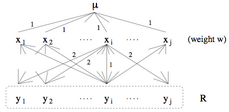## neal young / Chrobak06Reverse

•The Reverse Greedy algorithm (RGreedy) for the $$k$$-median problem works as follows. It starts by placing facilities on all nodes. At each step, it removes a facility to minimize the resulting total distance from the customers to the remaining facilities. It stops when $$k$$ facilities remain. We prove that, if the distance function is metric, then the approximation ratio of RGreedy is between $$\Omega(\log n/ \log \log n)$$ and $$O(\log n)$$.
Journal version of .